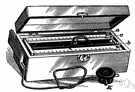# ohmmeter

Also found in: Thesaurus, Medical, Encyclopedia, Wikipedia.

## ohm·me·ter

(ōm′mē′tər)
n.
An instrument for measuring directly the resistances of conductors in ohms.

## ohmmeter

(ˈəʊmˌmiːtə)
n
(General Physics) an instrument for measuring electrical resistance

## ohm•me•ter

(ˈoʊmˌmi tər)

n.
an instrument for measuring electric resistance in ohms.
[1895–1900]

## ohmmeter

an instrument for measuring electrical resistance in ohms.
ThesaurusAntonymsRelated WordsSynonymsLegend:
 Noun 1ohmmeter - a meter for measuring electrical resistance in ohmsmeter - any of various measuring instruments for measuring a quantity
Translations
Mentioned in ?
References in periodicals archive ?
Tenders are invited for industrial insulation testers digital multi meter safety ohmmeter micro ohm meter
This procedure does not take long and does not require a controller, as a standard heat gun and ohmmeter will suffice.
Megger announced it recently donated a low-resistance ohmmeter to students in the Wind Energy and Turbine Technology and the Electrical Technology programs at Iowa Lakes Community College.
The continuity of these windings is critical to compressor operation, and can be tested using an ohmmeter to determine the health of each.
He let me use his ohmmeter to measure resistors, explaining to me how to read the color codes.
Then, using equation (7) the measured conductivity is measured as 1.1 x 10-5 ohmmeter ([OMEGA] - m).
Electrical resistivity of the electrodeposited films was measured using an ohmmeter (Fluke 1550B MegOhmMeter, USA).
The dimensions of electrodes determine factor k, ohmmeter measures resistance and surface resistivity [rho] is then calculated.
In the 5TE sensor, the soil conductance is independently determined from the voltage drop across two resistors in series with the soil, the functioning being similar to an ohmmeter. The [[sigma].sub.b] is then calculated from the soil conductance and the resistors' geometry.
Transepithelial resistance was measured in each well using a Millicell ERS ohmmeter (Millipore.
The pre-strike and poststrike measurements are made using a standard electrician's Ohmmeter, with a test voltage of 10 V DC.

Site: Follow: Share:
Open / Close